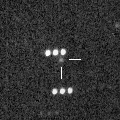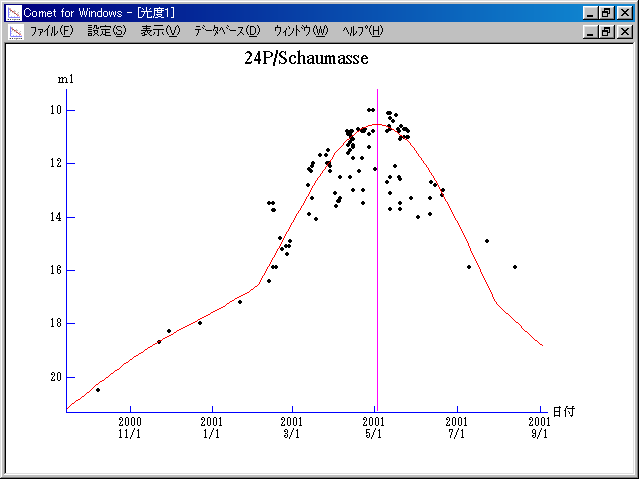# 24P/Schaumasse (2001)###Pictures###Orbital Elements

```   The following improved orbital elements by Kenji Muraoka,
are from 523 observations 1984 to 2001, including 9 Planets,
Moon and 5 minor planets perturbations and non-gravitational
effect of style II.  The mean residual is +/- 0.80 arc seconds.

Epoch  =  2001 May  11.0  TT       JDT = 2452040.5
T  =  2001 May   2.65966       +/- 0.00017 (m.e.) TT
Peri. =   57.87344                +/- 0.00010
Node  =   79.83119                +/- 0.00003   (2000.0)
Incl. =   11.75149                +/- 0.00001
q  =    1.2050046              +/- 0.0000004 AU
e  =    0.7048079              +/- 0.0000001
a  =    4.0821028              +/- 0.0000004 AU
ns =    0.11950283             +/- 0.00000002
P  =    8.248                  +/- 0.0000013  years
A1  =   +0.126                  +/- 0.014
A2  =   -0.05861                +/- 0.00007
```

###Finding Charts###Magnitudes Graph

```        m1 = 12.0 + 5 log d + 17.0 log r  [   ,-90]  (             - 2001 Feb.  1)
m1 =  6.0 + 5 log d + 45   log r  [-90, 90]  (2001 Feb.  1 - 2001 July 31)
m1 = 12.0 + 5 log d + 17.0 log r  [ 90,   ]  (2001 July 31  -            )
```##### The orbital elements are calculated by Kenji Muraoka. The charts are made with StellaNavigator Ver.2.0 for Windows (AstroArts / ASCII). The magnitudes graphs are made with Comet for Windows.